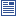Accessibility options:

# Mechanics resources

Show me all resources applicable to

### Community Project (1)Equations of motion (SOURCE)
This resource covering equations of constant acceleration has been contributed to the mathcentre Community Project by Josh Simpson and reviewed by Leslie Fletcher, Liverpool John Moores University.

### Motivating Mathematics (1)Force and motion - Mike Savage
This extension video mathstutor discusses Newton's second law of motion. This resource is released under a Creative Commons license Attribution-Non-Commercial-No Derivative Works and the copyright is held by Skillbank Solutions Ltd.

### Quick Reference (25)Conservation of momentum
This leaflet introduces the concept of conservation of momentum in both one and two dimensions.Constant acceleration equations
This leaflet deals with objects moving with constant acceleration.Conversion of units
On occasions it is necessary to convert from one system of units to another. This leaflet explains how this is done.Displacement-time and Velocity-time graphs
This leaflet explains how graphs can be used to describe mathematical models of motion.Equations of motion
This resource covering equations of constant acceleration has been contributed to the mathcentre Community Project by Josh Simpson and reviewed by Leslie Fletcher, Liverpool John Moores University.Equilibrium of a particle
This leaflet deals with resolving forces.Force as a Vector
This leaflet deals with forces as vectors.Force Diagrams
This leaflet deals with force diagrams.Forces acting at an angle - resolving forces
This leaflet explains how a force can be split into two perpendicular components.Forces acting at an angle (with friction)
This leaflet introduces friction into the analysis of the effect of forces on bodies.Friction
This leaflet introduces friction forces.Impact and momentum - definition and units
This leaflet introduces the concepts of impact and momentum.Introduction to mechanics
This leaflet explains the terms statics and dynamics and provides basic introductory material.Motion due to gravity
This leaflet introduces gravitational force.Newton's first law of motion
This leaflet introduces the first of the laws of motion.Newton's second law of motion
This leaflet introduces Newton's second law of motionNewton's third law of motion
This leaflet introduces Newton's third law of motion.Parallel forces acting together
In this leaflet we consider the resultant force when several parallel forces act.Particle on a slope (with friction)
This leaflet analyses particles on slopes in the presence of friction.Particles on a slope
When resolving forces, the directions generally taken are horizontal and vertical. However, there are situations when it is better to consider other directions for resolving. This leaflet explains how this is done.Pulleys
This leaflet considers problems involving pulleys.Resolving forces - i, j notation
This leaflet deals with resolving forces, using the I, j notation.Simplifying mechanics modeling
This leaflet deals with simplifying mechanics modeling.Types of Force
This leaflet explains the various types of force which are commonly found when studying the motion of physical bodies.Units and prefixes
This leaflet summarises the SI system of units and commonly used prefixes.